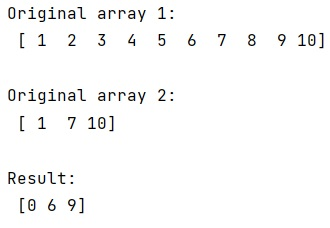# Find indices of matches of one array in another array

Learn, how to find indices of matches of one array in another array in Python? By Pranit Sharma Last updated : March 28, 2023

Suppose that we are given two numpy arrays, one contains unique values and another contains the same values as of first. We need to get the index of the second array's values within the first array.

## Finding indices of matches of one array in another array

To find indices of matches of one array in another array, we use numpy.in1d() with numpy.nonzero(). The numpy.in1d() is used to test whether each element of a 1-D array is also present in a second array. It returns a boolean array the same length as ar1 that is True where an element of ar1 is in ar2 and False otherwise.

Let us understand with the help of an example,

## Python code to find indices of matches of one array in another array

```# Import numpy
import numpy as np

# Creating numpy arrays
arr1 = np.array([1,2,3,4,5,6,7,8,9,10])
arr2 = np.array([1,7,10])

# Display Original arrays
print("Original array 1:\n",arr1,"\n")
print("Original array 2:\n",arr2,"\n")

# Getting second array's values
res = np.nonzero(np.in1d(arr1,arr2))

# Display result
print("Result:\n",res)
```

### Output